#Function Repository Resource:

# ExampleDataset

Retrieve example data as a dataset

Contributed by: Anton Antonov
 ResourceFunction["ExampleDataset"][arg] returns the ExampleData collection specified by arg as a Dataset.

## Details and Options

ResourceFunction["ExampleDataset"] has one argument that should be a viable first argument for ExampleData. Only data collections in the "MachineLearning" and "Statistics" domains are supported.

## Examples

### Basic Examples (2)

Get an example dataset:

 In:=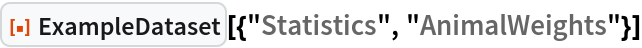Out=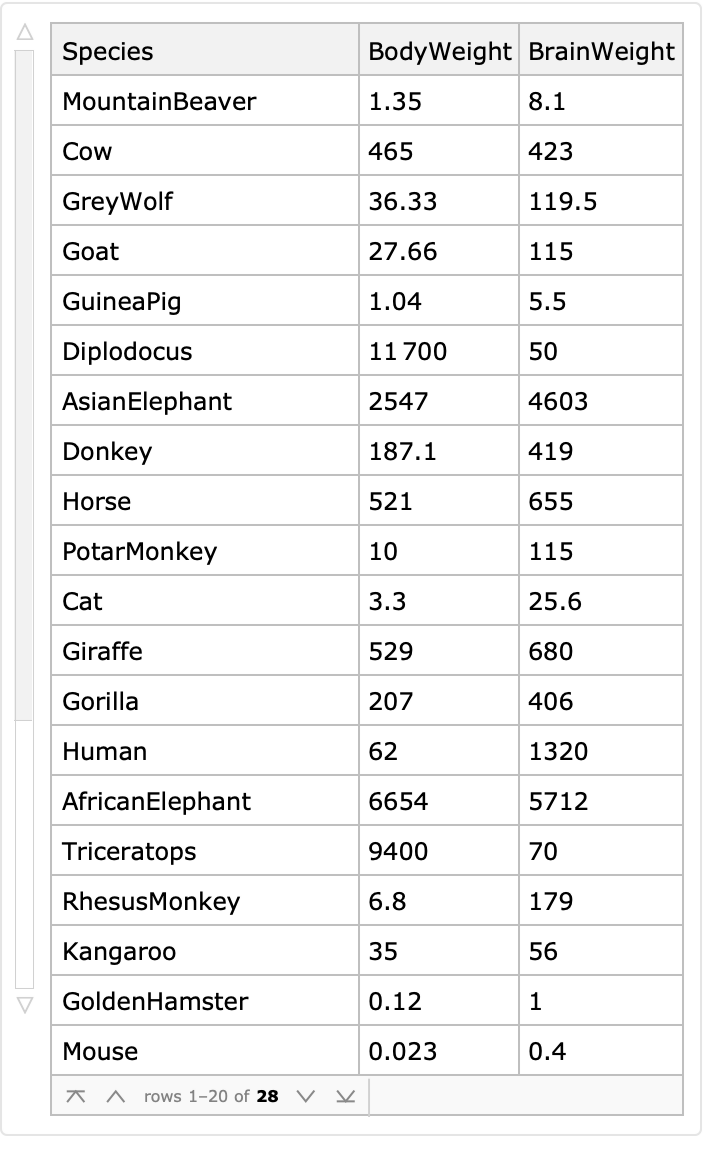Get another dataset:

 In:=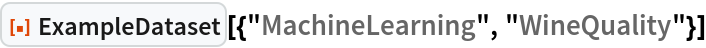Out=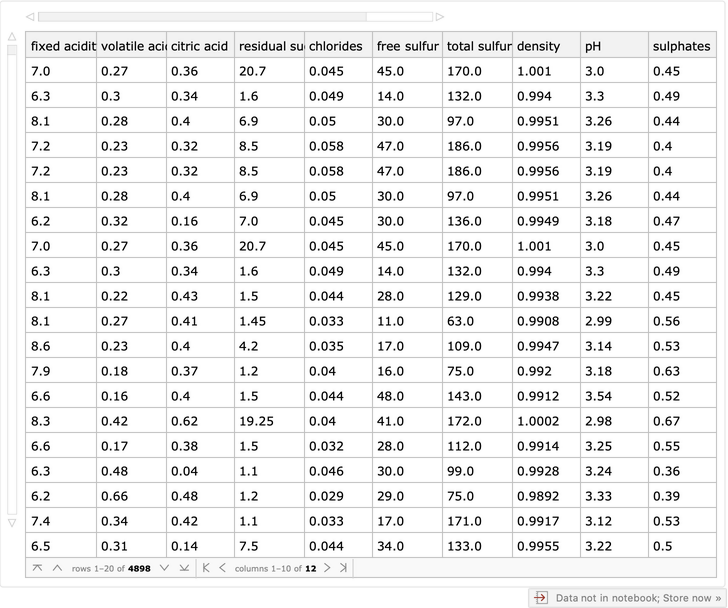### Applications (2)

Find homes in Boston with an age greater than 98 years:

 In:=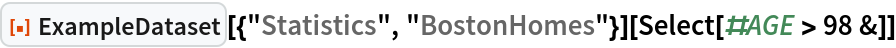Out=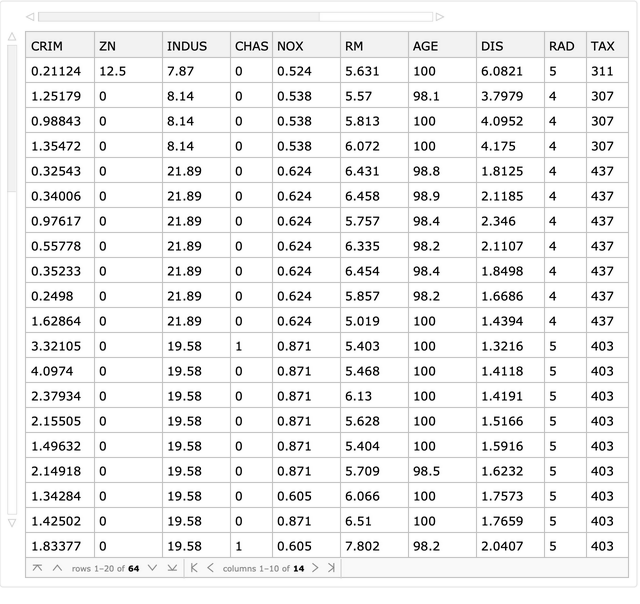Cross tabulate odor and edibility for mushrooms (we can see that odor is a good indicator of edibility):

 In:=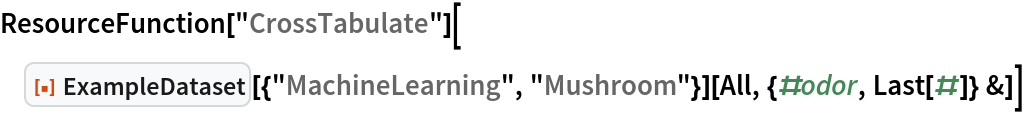Out=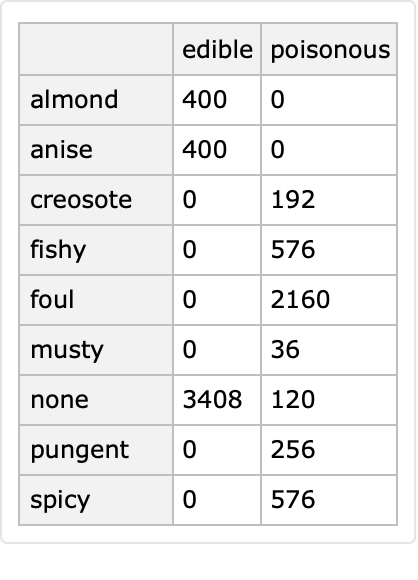### Possible Issues (3)

If an unknown dataset name is specified, then the result is a Failure:

 In:=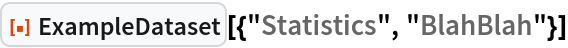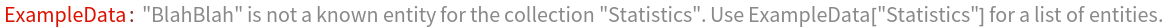Out=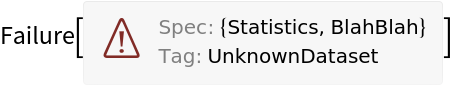The expected data types for the "Statistics" datasets in ExampleData are "MultivariateSample", "TimeSeries" and "EventSeries". ExampleData has the following data types:

 In:=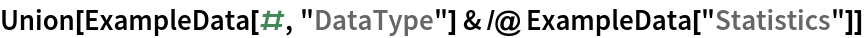Out=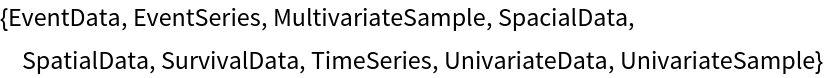A Failure is returned for other data types:

 In:=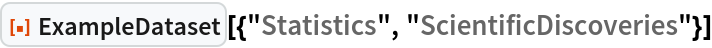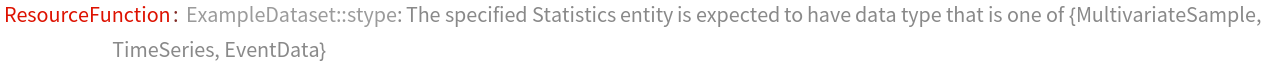Out=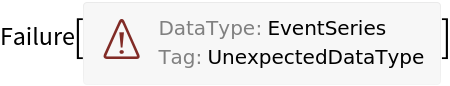Here is a summary of the successes and failures of for different data types in the "Statistics" example data collection:

 In:=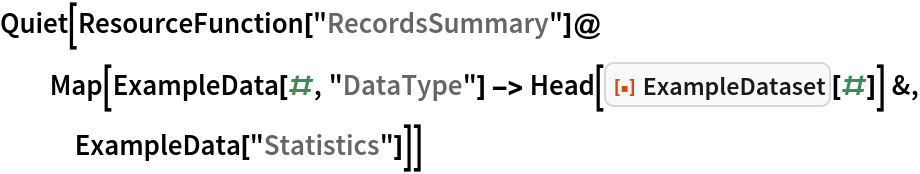Out=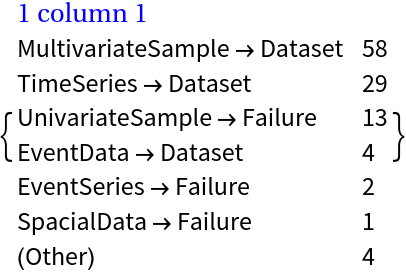Some "MachineLearnining" example data have data shapes and variable names that do not match. In those cases, ExampleDataset returns Failure:

 In:=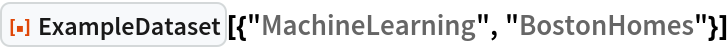Out=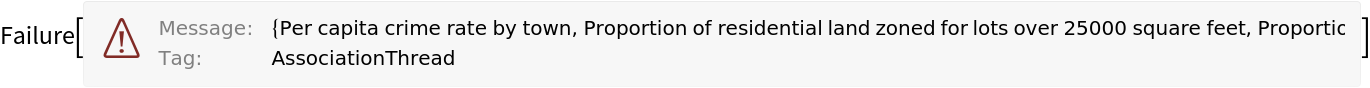Compare the length of the variable names:

 In:=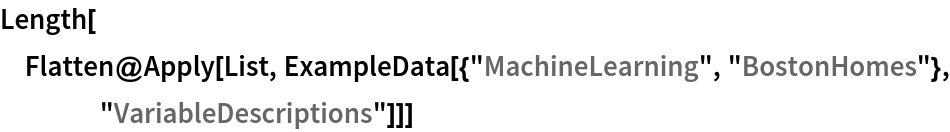Out=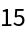With the dimensions of the data:

 In:=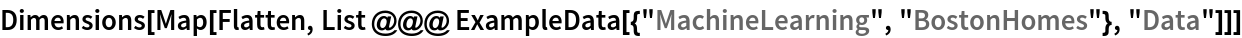Out=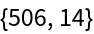Here is an association that shows the successes and failures over the "MachineLearning" datasets:

 In:=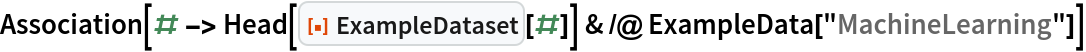Out=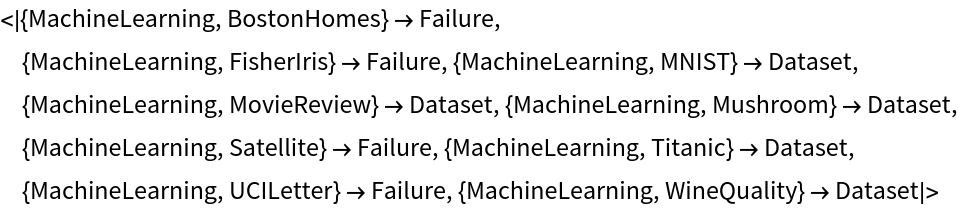### Neat Examples (1)

Summaries for all "Statistics" datasets in ExampleData that have six columns:

 In:=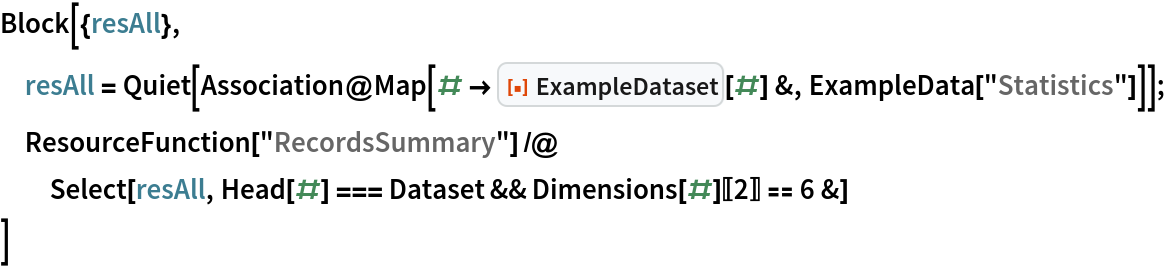Out=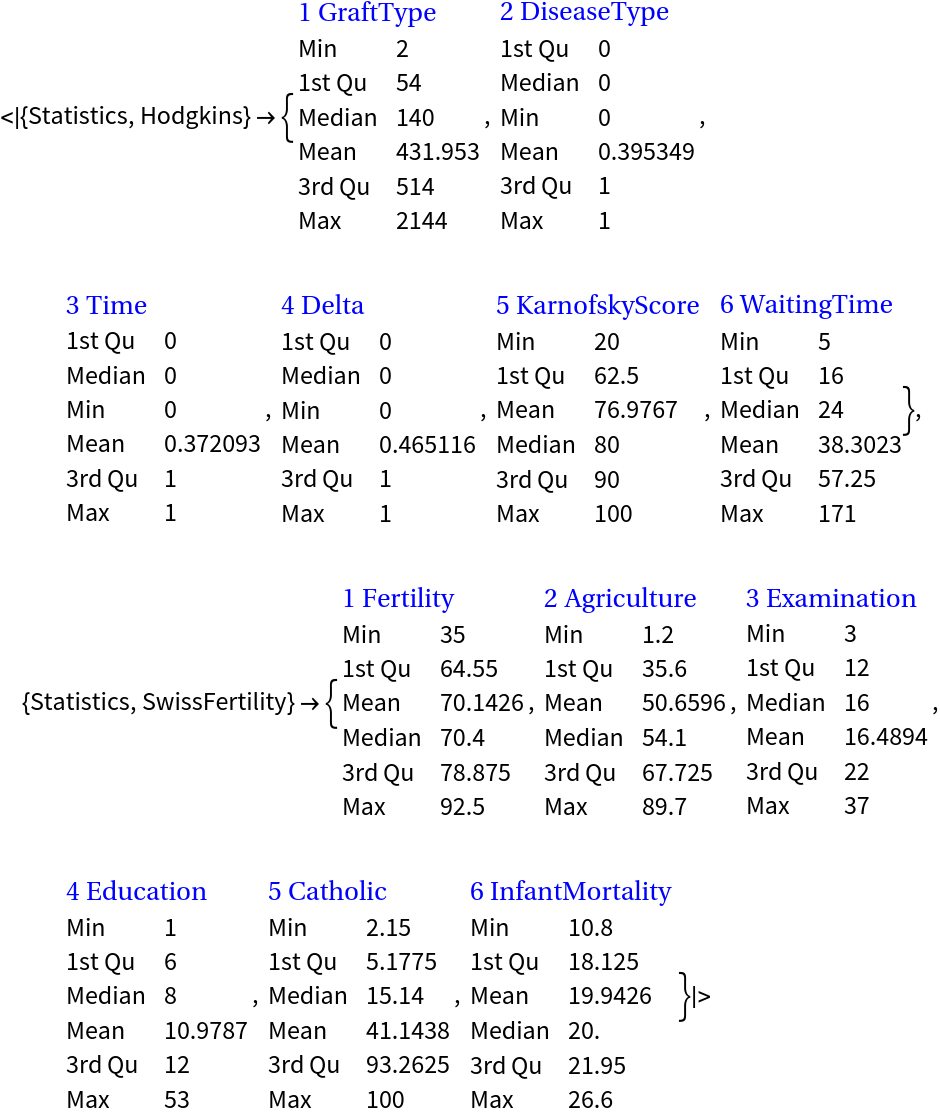## Author Notes

One way to implement the functionality of ExampleDataset is to use an overloading definition for ExampleData signatures. For example, we can have: ExampleData[spec,"Dataset"]. Alternatively, instead of overloading the unprotected symbol ExampleData, we can have a resource function.